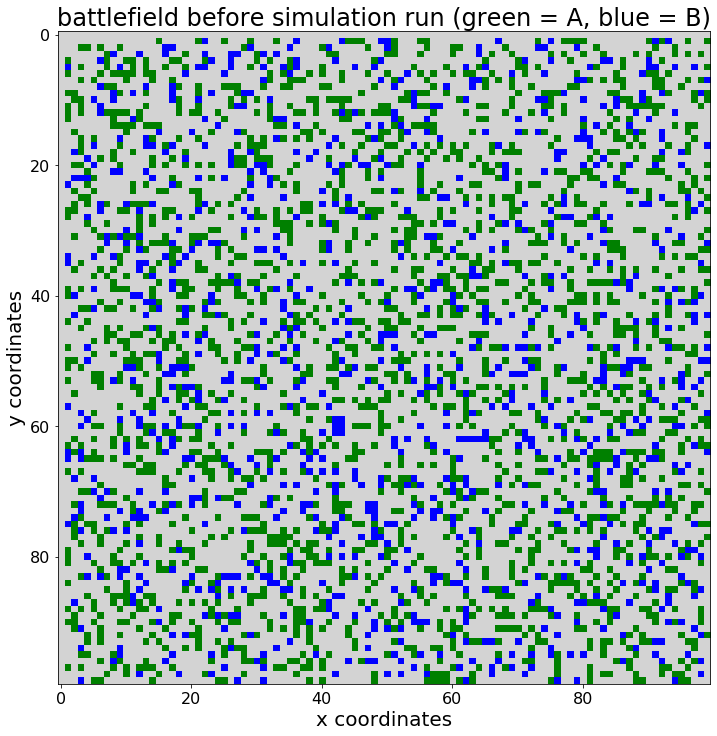# 用Python开发一个简单的基于代理的仿真模型```类，将代理定义为抽象的数据类型。
class agent:
# init方法，代理的构造方法
def __init__(self,x,y,group):
self.life = 100.0 # 命根子
self.x = x
self.y = y
self.group = group```

```# 在python中使用列表理解法创建空的100x100列表。
battlefield = [[None for i in range(0,100)] for i in range(0,100)]```

```# 列表中包含可用的X和Y位置
locations = battlefield.copy() # using .copy prevents copying by reference
# 创建空列表，以便将来包含代理参考，类型A和B
agents_A = []
agents_B = []
# 将随机点分配给A组和B组的代理。
# -- 为此我需要随机模块，所以导入它
import random
# -- 定义一个用于创建代理并将其分配到网格的功能。
def agentCreator(size,group,groupList,field,n,m):
# 循环往复
for j in range(0,size):
# 随机选址
while True:
# 随机座标
x = random.choice(range(0,n))
y = random.choice(range(0,m))
# 确认是否有空位，如果没有则重申
if field[x][y] == None:
field[x][y] = agent(x=x,y=y,group=group)
# 将代理对象引用添加到组列表中
groupList.append(field[x][y])
# 循环退出，就地取材
break
# -- 我使用agentCreator函数来填充战场。
agentCreator(size = 1000,
group = "A",
groupList = agents_A,
field = battlefield,
n = 100,
m = 100)
agentCreator(size = 1000,
group = "B",
groupList = agents_B,
field = battlefield,
n = 100,
m = 100) ```

2D列表 “战场 “现在包含 “无 “或代理人参考，类型为A或B，所有代理人的生命分值为100，因为战斗还没有开始。

```#.imshow()需要一个有浮动元素的矩阵。
population = [[0.0 for i in range(0,100)] for i in range(0,100)]
# if agent is of type A, put a 1.0, if of type B, pyt a 2.0
for i in range(1,100):
for j in range(1,100):
if battlefield[i][j] == None: # 虚空
pass # 在人口单元中留0.0
elif battlefield[i][j].group == "A": # 甲组代理人
population[i][j] = 1.0 # 1.0表示 "A"
else: # 乙类制剂
population[i][j] = 2.0 # 2.0意味着 "B"
# 从matplotlib导入pyplot和颜色。
from matplotlib import pyplot, colors
# 使用matplotlib中的颜色，定义一个颜色图。
colormap = colors.ListedColormap(["lightgrey","green","blue"])
# 用pyplot定义图形大小
pyplot.figure(figsize = (12,12))
# 用Pyplot加标题
pyplot.title("battlefield before simulation run (green = A, blue = B)",
fontsize = 24)
# 用pyplot添加x和y标签
pyplot.xlabel("x coordinates", fontsize = 20)
pyplot.ylabel("y coordinates", fontsize = 20)
# 使用pyplot调整x轴和y轴的刻度。
pyplot.xticks(fontsize = 16)
pyplot.yticks(fontsize = 16)
# 使用pyplot中的.imshow()方法来可视化代理的位置。
pyplot.imshow(X = population,
cmap = colormap)```
`<matplotlib.image.AxesImage at 0x1c756b04c88>`